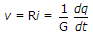# Electronics and Communication Engineering - Networks Analysis and Synthesis

1.

In the circuit of figure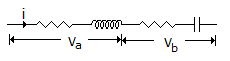A. Va would always lag Vb B. Va would always lead Vb C. Va and Vb can be in phase D. phase angle between Va and Vb would always be 90°

Explanation:

Voltage across inductance leads the current and voltage across capacitance lags the current.

2.

As per BIS, solid insulators are classified into 6 categories.

 A. True B. False

Explanation:

The number of categories is seven.

3.

Dielectric constant of air is about 5.

 A. True B. False

Explanation:

r for air is 1.

4.

Consider the following units

1. sec-1
3. sec
4. Ω
The units of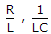, RC and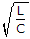are respectively

 A. 1, 2, 4, 3 B. 3, 2, 1, 4 C. 2, 4, 1, 3 D. 1, 2, 3, 4

Explanation: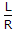has units of seconds. Therefore,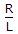has units of sec-1.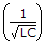has units of rad/sec.

Therefore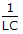has units of rad2/sec2, RC is a time constant and has units of sec.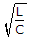has units of ohms.

5.

In a conductor, voltage v, conductance G and charges q are related as

 A.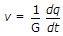B.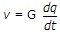C. v = Gq D. q = vG